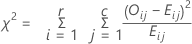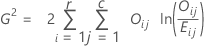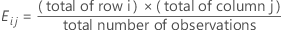Methods and formulas for chi-square test for association for Cross Tabulation and Chi-Square

Select the method or formula of your choice.

Chi-square test

Formula

Minitab displays two chi-square statistics.

Pearson chi-square statistic:Likelihood-ratio chi-square statistic:Note

If any cell has an expected frequency less than one, the p-value for the test is not displayed because the results may not be valid. If some cells have small expected frequencies, consider combining or omitting row and/or column categories.

Yates' correction for 2 x 2 tables is not used.

Notation

TermDescription
Oijobserved frequency in cell (i, j)
Eij

expected frequency for cell (i, j)Expected cell count

The expected count is the frequency that would be expected in a cell, on average, if the variables are independent. Minitab calculates the expected counts as the product of the row and column totals, divided by the total number of observations.

Notation

TermDescription
ni+ number of observations in the ith row
n+j number of observations in the jth column
n++ total number of observations

Raw residual calculation

Formula

Raw residual = Oij – Eij

Notation

TermDescription
Oij observed frequency in cell (i, j)
Eij expected frequency for cell (i, j)

Standardized residual calculation

Notation

TermDescription
Oij observed frequency in cell (i, j)
Eij expected frequency for cell (i, j)

Notation

TermDescription
Oij observed frequency in cell (i, j)
Eij expected frequency for cell (i, j)
ni+ number of observations in the ith row
n+j number of observations in the jth column
n++ total number of observations

Contribution to chi-square statistic from each cell

Formula

The contribution to the χ2 statistic from each cell is:

Notation

TermDescription
Oij observed frequency in cell (i, j)
Eij expected frequency in cell (i, j)
By using this site you agree to the use of cookies for analytics and personalized content.  Read our policy